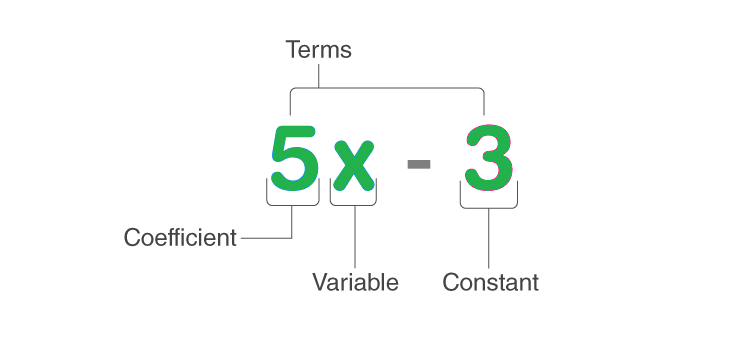Open in App
Not now

# Prove that the square of any natural number is either a multiple of 3 or one more than a multiple of 3

• Last Updated : 22 Sep, 2021

Number System is a system of representing numbers using symbols and digits in a certain manner. One can think of it as a Grammar book in mathematics. As soon as one hear this word “Number” 1,2,3,…. get pop in one’s head immediately. Number System defines their value, operations to perform, and other properties. Each number is unique. It has many variations. Thus, it can be considered as a natural number, whole number, even number, odd number, prime number, composite number, etc.

1. Natural Number – It contains numbers starting from 1.
2. Whole Number – It contains numbers starting from 0.
3. Even Number – Numbers that are divisible by 2.
4. Odd Number – Numbers that are not divisible by 2.
5. Prime Number – Numbers that are divisible by 1 and itself only. i.e. only two factors.
6. Composite Number – Numbers that are divisible by 1and itself and others. i.e. more than two factors.

### Algebra

Algebra is the branch of Mathematics that emphasizes the use of various symbols and mathematical operations that can be performed on them. In Algebra, symbols such as alphabets are used like a, b, c or x, and many others whose value is not predefined. Their value is not fixed, hence called variables. One can declare any amount of variables and define the specific value to them. These symbols or variables can be manipulated with help of arithmetic operations like addition, subtraction, multiplication, and division. Let us deal with one application of Algebra which is Algebraic Expression.An algebraic expression is composed of coefficients, Terms, Variables, and Constant, etc. and people have to determine the value of an unknown variable with mathematical operations.

### Natural Numbers

Natural numbers are the counting numbers that begin from 1 till infinity, They are used for counting purposes. e.g. 1,2,3,4,…. , are natural numbers. Basically, Natural numbers are an integral part of the real number system.

However, Natural numbers doesn’t include zero, fraction, decimal, negative numbers.

A set of Natural numbers is denoted by N such that N = {1, 2, 3, 4, 5, 6, 7, 8,….}.

### Use algebra to prove that the square of any natural number is either a multiple of 3 or one more than a multiple of 3.

Solution:

Suppose, we have a natural number  N.

Consider three cases where N is in form of  3*N, 3*N+1, 3*N+2.

Squaring, we get

• The square of 1st term is 9N2  which is a multiple of 3.
• Square of 2nd term is:

9N2 + 6N +1 = 3(3N2 + 2N) + 1 which is one more than a multiple of 3.

• Square of 3rd term is:

9N2 + 12N + 4 =  9N2 +12N + 3 + 1 = 3(3N2 + 4N + 1) +1 which is one more than multiple of 3.

Hence, it can be said that for N2  to be multiple of 3, N should be in form of 3N, 3N+1, 3N+2, and so on.

Example:

Hence, the square of any natural number is either a multiple of 3 or one more than a multiple of 3.

### Similar Problems

Question 1: Prove that the sum of two consecutive square numbers is odd?

Solution:

Let us assume that N and N + 1 are two consecutive numbers where N is any integer.

We have to determine their sum. So,

Sum = N2 + (N + 1)2 = N2 + N2 + 2N + 1 = 2N2 + 2N + 1 = 2(N2 + N) +1

The definition of odd number is one more than multiple of 2.

The sum is one more than multiple of 2. So, their sum is odd.

Example:

Input N = 2 and N + 1 = 3

Output 22 + 32 = 4+ 9 = 13

Conclusion The sum is odd.

Question 2: Prove that if m is not the square of a natural number, then √m is irrational.

Solution:

Let m be any positive integer such that there is no m = x2 where x is an integer.

We assume that √m is a rational number. Then it can be written as:

√m = p/q  ⇢ (1)

Where p and q have no common factor other than 1.

Squaring both sides of Equation (1)

m = p2 / q2.

Since n is a positive integer and p and q have no common factor besides (1). Let q = 1.

m = p2

This result is contradictory to our assumption that m is not a square of a number. Hence, √m is irrational.

Question 3: Prove that if n is a natural number, then √n is irrational or a natural number.

Solution:

Let n be any natural number.

Case 1: n is a perfect square (1, 4, 9, 16, etc.)

Hence, √n is a natural number.

Case 2: n is not a perfect square (√2, √3, √7, etc.)

Hence, √n is an irrational number.

Conclusion √n is either a natural number or an irrational number.

Question 4: Prove that product of two perfect square numbers is also a perfect square.

Solution:

Let p and q be any two positive integers.

Since both p and q are perfect squares, we can write

p = a2

q = b2

Where a and b are any two integers.

p × q = a2 × b2 = (a × a) × (b × b) = (a × b) × (a × b) = (ab) × (ab) = (ab)2

Hence, The result is a perfect square of ab.

Conclusion The product of two perfect square numbers is also perfect square.

Example:

Input p=4 and q=9

Output pq= 4*9 = 36 =62

Which is a perfect square.

My Personal Notes arrow_drop_up